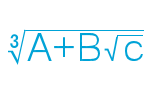# Nested roots, part 2I have recently came across a problem involving cube roots of numbers added to square roots, in the form of $$\sqrt{X+Y\sqrt{Z}}$$, this is called a “nested radical.” I already figured out a nested square root, I decided to give this a go.

I can take a number like $$(1+\sqrt5)^2$$ and work out it is equal to $$16 + 8\sqrt5$$. Unfortunately, it is very difficult to recognize that $$1+\sqrt5$$ is the cube root (or even a factor) of that. Even harder, trying to find an equation such as $$\sqrt{1080-624\sqrt3}$$.

Recently, I developed a method of breaking down the equation into its cube root equation.

With an equation in the form of $$\sqrt{X+Y\sqrt{Z}}$$, where Z contains no perfect square factors.

\begin{align} \sqrt{X+Y\sqrt{Z}} &= A+B\sqrt{Z} \\ X+Y\sqrt{Z} &= (A+B\sqrt{Z})^3 \\ &=A^3 + 3A^2B\sqrt{Z} + 3AB^2Z + B^3Z\sqrt{Z} \\ &=A^3 + 3AB^2Z + 3A^2B\sqrt{Z} + B^3Z\sqrt{Z} \\ &=\color{Yellow}{A^3 + 3AB^2Z} +\color{cyan}{( 3A^2B + B^3Z})\sqrt{Z} .\\ \end{align}

We need to find
\begin{align} X &= \color{Yellow}{A^3 + 3AB^2Z} \tag{1}\label{1} \\ Y &=\color{cyan}{3A^2B + B^3Z}. \tag{2}\label{2}\\ \end{align}

If You look at X, You see it is the sum of a cube and a multiple of 3Z.

Let’s look at an example, $$\sqrt{1080-624\sqrt{3}}$$. If we subtract cubes from the initial number(1080) we get:

\begin{align} 1080-1^3 &= 1080-1= 1079 &\color{red}{No} \\ 1080-2^3 &= 1080-8= 1072 &\color{red}{No}\\ 1080-3^3 &= 1080-27=1053=3Z\cdot 117 &\color{lime}{Yes} \\ 1080-4^3 &= 1080-64=1016 &\color{red}{No}\\ 1080-5^3 &= 1080-125=955 &\color{red}{No}\\ 1080-6^3 &= 1080-216=864=3Z\cdot 96 &\color{lime}{Yes} \\ 1080-7^3 &= 1080-343=737 &\color{red}{No}\\ 1080-8^3 &= 1080-512=568 &\color{red}{No}\\ 1080-9^3 &= 1080-729=351=3Z\cdot 39 &\color{lime}{Yes} \\ 1080-10^3 &= 1080-1000=80 &\color{red}{No}\\ 1080-11^3 &= 1080-1331=-251 &\color{red}{No}\\ \end{align}

We need a result that is evenly divisible by 3Z, or 9 in this case. $$11^3$$ is greater than 1080, so we stop. (Even if all answers are complex, will still be Real.)

Our results show 3, 6, and 9 are viable solutions for a, we must now check each against X to get B.

 if(A=3) $$1053 =9\cdot 3\cdot B^2$$ B²=39 $$B=\sqrt{39}$$ if(A=6) $$864 =9\cdot 6\cdot B^2$$ B²=16 $$B=4$$ if(A=9) $$351 =9\cdot 9\cdot B^2$$ $$B^2=4\frac13$$ $$B=\sqrt{\frac{13}{3}}$$

The only even square root is for A=6, B=4. $$X= 6^3 + 3\cdot6 \cdot 4^2 \cdot 3 =1080$$, so this is a possible solution pair. First we must now check against Y.

\begin{align} Y &=3A^2B + B^3Z\\ &=3\cdot 6^2\cdot4 + 4^3\cdot3\\ &=3\cdot 36\cdot4 + 64\cdot3\\ &=432 + 192\\ &=624\\ \end{align}

It matches, so we have a correct solution for $$\sqrt{1080-624\sqrt{3}} =6-4\sqrt{3}$$. Remember, as the original had a negative Y, the solution must negate B.

If our example $$\sqrt{1080-624\sqrt{3}}$$ was actually $$\sqrt{1080-623\sqrt{3}}$$, then the formulas above would not work (there would not be any solution) and would not be able to be simplified any further.

Let’s try $$\sqrt{\frac{371}{125}+\frac{319}{100}\sqrt2 }$$, which can also be written $$\sqrt{2.968+3.19\sqrt2 }$$. This is a bit harder as we are not dealing with integers. It may be easier to try smaller cubes.

\begin{align} 2.968-.1^3 &= 2.968-.001= 2.967=3Z\cdot 0.4945 \\ 2.968-.2^3 &= 2.968-.008= 2.960\\ 2.968-.3^3 &= 2.968-.027= 2.941\\ 2.968-.4^3 &= 2.968-.064= 2.904=3Z\cdot 0.4840\\ 2.968-.5^3 &= 2.968-.125= 2.843\\ 2.968-.6^3 &= 2.968-.216= 2.752\\ 2.968-.7^3 &= 2.968-.343= 2.625=3Z\cdot 0.4375\\ 2.968-.8^3 &= 2.968-.512= 2.456\\ 2.968-.9^3 &= 2.968-.729= 2.239\\ 2.968-1.0^3 &= 2.968-1.000= 1.968=3Z\cdot 0.328\\ 2.968-1.1^3 &= 2.968-1.331= 1.637\\ 2.968-1.2^3 &= 2.968-1.728= 1.240\\ 2.968-1.3^3 &= 2.968-2.197= 0.771=3Z\cdot 0.1285\\ 2.968-1.4^3 &= 2.968-2.744= 0.224\\ 2.968-1.5^3 &= 2.968-3.375=-0.407 \\ \end{align}

Of these, 0.1, 0.4, 0.7, 1, and 1.3 are finitely divisible by 6 (3Z). If we didn’t find any, we would have to try smaller numbers.

 if (A=0.1) $$2.967=6\cdot 0.1\cdot B^2$$ $$B^2=\frac{4945}{1000}$$ B is irrational if (A=0.4) $$2.904=6\cdot 0.4\cdot B^2$$ $$B^2=\frac{121}{100}$$ $$B=\frac{11}{10}$$ if (A=0.7) $$2.625=6\cdot 0.7\cdot B^2$$ $$B^2=\frac58$$ $$B=\sqrt{\frac58}$$ if (A=1) $$1.968=6\cdot 1\cdot B^2$$ $$B^2=\frac{41}{125}$$ B is irrational if (A=1.3) $$0.771=6\cdot 1.3\cdot B^2$$ $$B^2=\frac{257}{2600}$$ B is irrational

Three of these are obviously irrational, so cannot be part of our solution, so are not calculated.

For A=0.7, B is also irrational, so we will first test A=0.4:

\begin{align} 2.968 &= A^3 + 3AB^2Z\\ &=0.4^3 +3\cdot 0.4\cdot B^2\cdot 2\\ &=0.064 + 2.4\cdot B^2\\ 2.904&=2.4B^2\\ 1.21&=B^2\\ B&=\frac{11}{10}=1.1\\ \end{align}

And for Y:

\begin{align} Y &=3A^2B + B^3Z\\ &=3\cdot 0.4^2\cdot1.1 + 1.1^3\cdot2\\ &=3\cdot 0.16\cdot1.1 + 1.331\cdot2\\ &=0.528 + 2.662\\ &=3.19\\ \end{align}

It matches, so we have a correct solution for $$\sqrt{2.968+3.19\sqrt2 } =0.4+1.1\sqrt{2}$$.

Any cube root in this form must also have $$X^2+Y^2\cdot Z$$ equal a perfect cube.

$$\sqrt{1080^2-624^2\cdot 3}= -12$$ and $$\sqrt{\frac{371}{125}^2-\frac{319}{100}^2\cdot2}=\frac{-113}{50}$$, so these are solvable.

Also, remember that these are cube roots, so there are two complex roots. Just rotate the answer found by 120° and 240°, for the remaining answers. If this technique doesn’t find a simple answer, then all 3 may be complex.

Another way to solve, if Z=5 and the number under the radical is in the form of $$2K\pm K\sqrt5$$, aka $$K\cdot(2\pm \sqrt5)$$, the answer will be in the form of $$A\pm +A\sqrt5$$. In this case, $$A=\sqrt{\frac{K}{8}}$$.

Alternate values for Z:

 Z Form A= 2 $$K(7\pm 5\sqrt2)$$ $$\sqrt{K}$$ 3 $$K(5\pm 3\sqrt3)$$ $$\sqrt{\frac{K}{2}}$$ 5 $$K(2\pm \sqrt5)$$ $$\sqrt{\frac{K}{8}}$$ 6 $$K(19\pm 9\sqrt6)$$ $$\sqrt{K}$$ 7 $$K(11\pm 5\sqrt7)$$ $$\sqrt{\frac{K}{2}}$$ 8 $$K(25\pm 11\sqrt8)$$ or $$K(25\pm 22\sqrt2)$$ $$\sqrt{K}$$ 10 $$K(31\pm 13\sqrt{10})$$ $$\sqrt{K}$$

This is likely not the best method for finding the cube roots, but it does work.